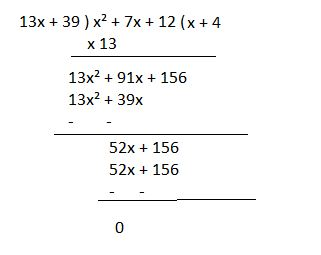# Worksheet on H.C.F. of Polynomials | Highest Common Factor of Two or More Polynomials Worksheet |

Students can practice various questions regarding the highest common factor of polynomials to get a grip on the topic. This Worksheet on H.C.F. of Polynomials includes all problems and solutions. Observe the simple steps to solve the greatest common factor of two or more polynomials using the factorization and division method on this page. We are advising you to practice the questions on your own and cross-check your answers with the step by step solutions provided to understand where you went wrong.

1. Find the greatest common factor (G.C.F) of two polynomials:

(a) 9u³(u⁴ – 2v³ + 1)², 6u⁴v⁵(u⁴ – 2v³ + 1)³

(b) 15x³yz²(y + z), 9xy⁴z³(2x + y + z)

(c) x⁴ – 27a³x, (x – 3a)²

(d) 2x² + 9x + 4, 2x² + 11x + 5

Solution:

(a) 9u³(u⁴ – 2v³ + 1)², 6u⁴v⁵(u⁴ – 2v³ + 1)³

Factorize the given two polynomials,

3u³(u⁴ – 2v³ + 1)² = 3x3xuxuxu (u⁴ – 2v³ + 1)(u⁴ – 2v³ + 1)

6u⁴v⁵(u⁴ – 2v³ + 1)³ = 3x2xuxuxuxuxvxvxvxvxv (u⁴ – 2v³ + 1)(u⁴ – 2v³ + 1)(u⁴ – 2v³ + 1)

Therefore, in the two polynomials, the common factors are 3 x uxuxu (u⁴ – 2v³ + 1)(u⁴ – 2v³ + 1)

So, the H.C.F = 3u³(u⁴ – 2v³ + 1)²

(b) 15x³yz²(y + z), 9xy⁴z³(2x + y + z)

Factorize the given two polynomials,

15x³yz²(y + z) =3*5*x*x*x*y*z*z (y + z)

9xy⁴z³(2x + y + z) = 3*3*y*y*y*y*z*z*z (2x + y + z)

Therefore, in two polynomials, the common factors are 3*x*y*z*z = 3xyz²

So, the required greatest common factor = 3xyz²

(c) x⁴ – 27a³x, (x – 3a)²

Factorize the given two polynomials,

x⁴ – 27a³x = x(x³ – 27a³) = x(x³ – (3a)³)

= x(x – 3a) (x²+3ax+9a²) i.e (a³ – b³) = (a – b) (a² + ab + b²)

(x – 3a)² = (x – 3a)(x – 3a)

So, in two polynomials the common factor is (x-3a).

Therefore, the required highest common factor = (x – 3a).

(d) 2x² + 9x + 4, 2x² + 11x + 5

By factorizing the two polynomials,

2x² + 9x + 4 = 2x² + 8x + x + 4

= 2x(x + 4) +1(x + 4) = (2x+1)(x+4)

2x² + 11x + 5 = 2x² + 10x + x + 5

= 2x(x+5) +1(x+5) = (2x+1)(x+5)

So, in both polynomials, the common factor is (2x+1).

Therefore, required G.C.F = (2x+1).

2. Find the highest common factor (H.C.F) of three polynomials:

(a) (s²-25t²)⁹, (s+5t)⁸, s²+10st+25t²

(b) 84a⁴b⁶(a⁴-b⁴), 92ab⁷(a²+b²)², 66a⁸b²

(c) 3x⁴ + 8x³ + 4x², 3x⁵ + 11x⁴ + 6x³ and 3x⁴ – 16x³ – 12x³

(d) 4y²-y-18, (4y-9)⁴, 4y-9

Solution:

(a) (s²-25t²)⁹, (s+5t)⁸, s²+10st+25t²

Factorize all three polynomials,

(s²-25t²)⁹ = (s² – (5t)²)⁹ = [(s+5t) (s-5t)]⁹

= (s+5t)⁹ (s-5t)⁹

(s+5t)⁸ = (s+5t) (s+5t) (s+5t) (s+5t) (s+5t) (s+5t) (s+5t) (s+5t)

s²+10st+25t² = s²+5st+5st+25t²

= s(s+5t) +5t(s+5t) = (s+5t) (s+5t)

So, in all polynomials, the common factor is (s+5t) (s+5t) = (s+5t)²

Therefore, required G.C.F = (s+5t)²

(b) 84a⁴b⁶(a⁴-b⁴), 92ab⁷(a²+b²)², 66a⁸b²

84a⁴b⁶(a⁴-b⁴) = 2x2x3x7xaxaxaxaxbxbxbxbxbxbx((a²)²-(b²)²)

= 2x2x3x7xaxaxaxaxbxbxbxbxbxbx(a²-b²)(a²+b²)

= 2x2x3x7xaxaxaxaxbxbxbxbxbxbx(a+b)(a-b)(a²+b²)

92ab⁷(a²+b²)² =2x2x23xaxbxbxbxbxbxbxbx(a²+b²)(a²+b²)

66a⁸b² = 2x3x11xaxaxaxaxaxaxaxaxbxb

So, the common factors in all polynomials is 2xaxbxb = 2ab²

Therefore, the required G.C.F is 2ab².

(c) 3x⁴ + 8x³ + 4x², 3x⁵ + 11x⁴ + 6x³ and 3x⁴ – 16x³ – 12x³

Factorizing all the polynomials,

3x⁴ + 8x³ + 4x² = x²(3x² + 8x + 4)

= x²(3x² + 6x + 2x + 4) = x²(3x(x + 2) +2(x + 2)

= x²(3x + 2) (x + 2)

3x⁵ + 11x⁴ + 6x³ = x³(3x² + 11x + 6)

= x³(3x² + 9x + 2x + 6)

= x³(3x(x + 3) + 2(x +3)

= x³(3x + 2) (x + 3)

3x⁴ – 16x³ – 12x³ = x²(3x² – 16x – 12)

= x²(3x² – 18x + 2x – 12) = x²(3x(x – 6) + 2(x – 6))

= x²(3x + 2) (x – 6)

So, the common factors are x²(3x + 2)

Therefore, the required highest common factor is x²(3x + 2).

(d) 4y²-y-18, (4y-9)⁴, 4y-9

Factorize all polynomials,

4y²-y-18 = 4y²-9y+8y-18

= y(4y-9)+2(4y-9)

= (y+2) (4y+9)

(4y-9)⁴ =  (4y+9) (4y+9) (4y+9) (4y+9)

4y-9 = (4y+9)

So, the common factor is (4y-9).

Therefore, the required greatest common factor is (4y+9).

3. Find the H.C.F of the below-mentioned polynomials by using the division method:

(a) 5x³-10x²-65x-50, 5x³-25x²-5x+25

(b) x³-x²-2x+2, x³-1

(c) x²+7x+12, 13x⁵+39x⁴

Solution:

(a) 5x³-10x²-65x-50, 5x³-25x²-5x+25

Given polynomials are f(x) = 5x³-10x²-65x-50, g(x) = 5x³-25x²-5x+25

Take 5 common from both polynomials, f(x) = 5(x³-2x²-13x-10), g(x) = 5(x³-5x²-x+5)

By using the division method,Thus, H.C.F of x³-2x²-13x-10, x³-5x²-x+5 = (x-2)

Therefore, G.C.F of 5x³-10x²-65x-50, 5x³-25x²-5x+25 = 5 (x – 2)

(b) x³-x²-2x+2, x³-1

Given polynomials are f(x) = x³-x²-2x+2, g(x) = x³-1

Arranging the polynomials in the descending order g(x) = x³+0x²+0x-1

By using the division method,Thus, H.C.F of x³-x²-2x+2, x³-1 is (x – 1).

(c) x²+7x+12, 13x⁵+39x⁴

Given polynomials are f(x) = x²+7x+12, g(x) = 13x⁵+39x⁴

Taking x⁴ common in g(x) i.e g(x) = x⁴(13x + 39)

By using the division method,4. Calculate the G.C.F of polynomials:

(a) 18x⁴-36x³+18x², 45x⁶-45x³

(b) k² – x², k² – kx and k²x – kx²

Solution:

(a) 18x⁴-36x³+18x², 45x⁶-45x³

Factorize the polynomials,

18x⁴-36x³+18x² = 18x² (x² – 2x + 1)

= 18x² (x² – x – x + 1)

= 18x² (x(x – 1) -1 (x – 1)) = 18x² (x – 1) (x – 1)

45x⁶-45x³ = 45x³(x³ – 1)

= 45x³(x – 1) (x² + x + 1)

Thus, H.C.F of 18x⁴-36x³+18x², 45x⁶-45x³ = 9x²(x – 1).

(b) k² – x², k² – kx and k²x – kx²

Factorize the polynomials,

k² – x² = (k – x) (k + x)

k² – kx = k(k – x)

k²x – kx² = kx(k – x)

Thus, G.C.F of k² – x², k² – kx and k²x – kx² = (k – x).